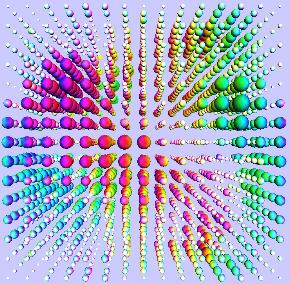#### R. Babich, J. Howard, C. Rebbi, Department of Physics and Center for Computational Science, Boston University, USA C. Hoelbling, Department of Physics, Bergische Universitat Wuppertal, Germany N. Garron, L. Lellouch, S. Necco, Centre de Physique Theorique, Marseille, France F. Berruto, Department of Physics, Brookhaven National Laboratory, USA N. Shoresh, Bauer Center for Genomics Research, Harvard University, USAThe figure illustrates the “probability amplitude” to find the quark and antiquark in a meson r lattice spacings apart after a time t (in lattice units) of propagation.

Quantum ChromoDynamics explains the structure of nuclear particles in terms of quarks held together by gluons. To perform numerical calculations quarks and gluons are placed on the sites and links of a space-time lattice.

The propagation of quarks in space-time is described by the Dirac equation. The recently proposed overlap formulation of the Dirac equation on a space-time lattice preserves chiral symmetry, a crucial property of QCD that was lost in earlier lattice discretizations, but comes at a heavy computational cost: one must calculate

Μ-1/2 Ψ

where Μ is a 4.5M × 4.5M complex matrix and ψ is the quark field. Special numerical techniques are used to evaluate this expression and thus the propagation of quarks over the space-time lattice, from which one can then calculate many properties of QCD.

As the quark and antiquark propagate in time the “amplitude” settles into the “wave-function” of a meson (from research by R. Babich, F. Berruto, N. Garron, C. Hoelbling, J. Howard, L. Lellouch, C. Rebbi and N. Shoresh, using Boston University’s IBM p690 server, partially supported by the US Department of Energy).The figure visualizes a quark field after 10 time units of propagation since the quark was created on the lattice.

#### Software for Lattice QCD

The MILC code, a specialized software package for lattice QCD simulations, has been ported to Boston University’s IBM eServer Blue Gene Solution. With some optimization, the code achieves a performance of close to 1TFlops sustained (work by J. Osborn, under the auspices of the SciDAC program of the US Department of Energy).